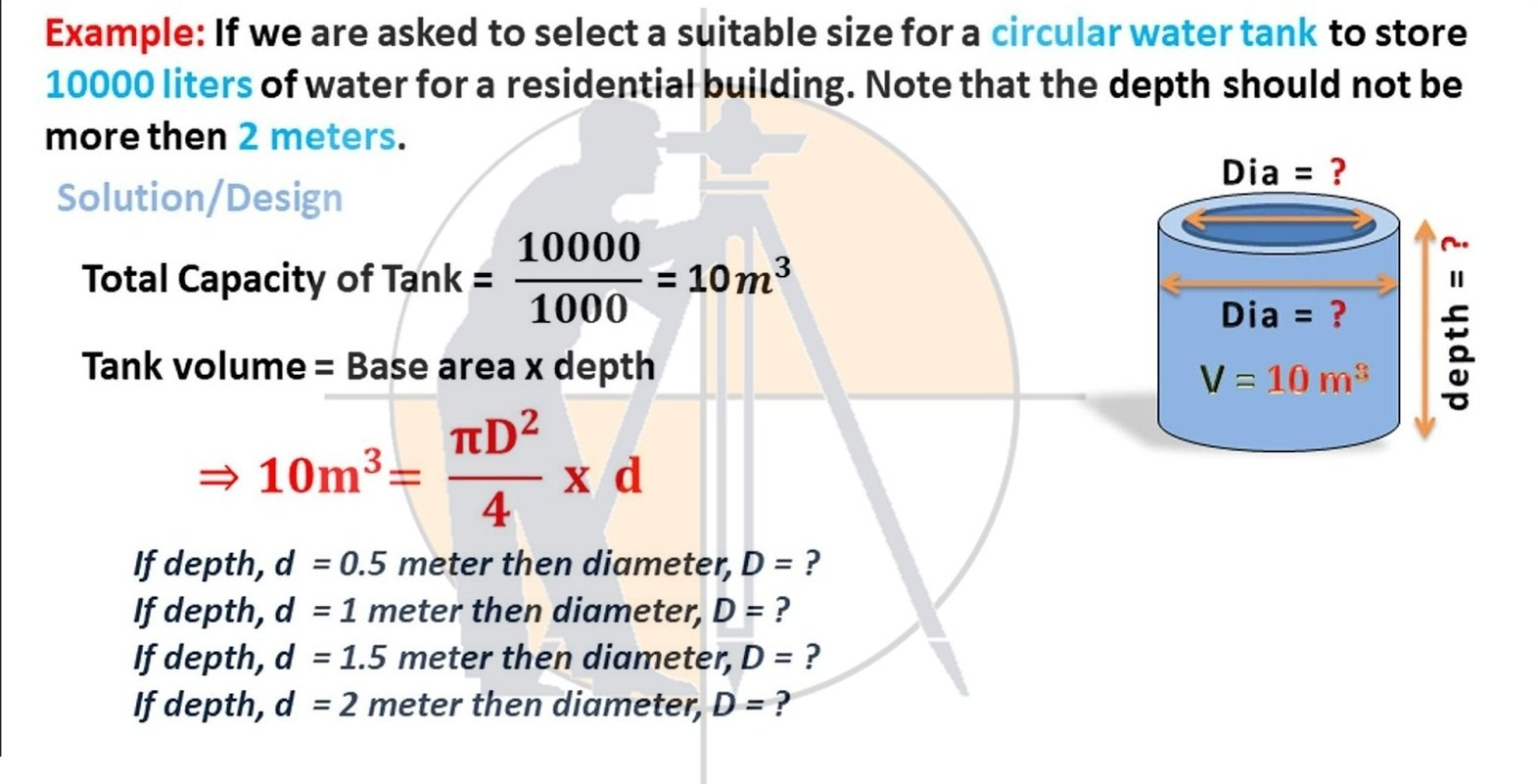Breaking News
Home / QA/QC Engineering / 10000 Liter Capacity Circular Water Tank Design Calculation With Full Detail

# 10000 Liter Capacity Circular Water Tank Design Calculation With Full Detail

### 10000 Liter Capacity Circular Water Tank Design Calculation With Full Detail

If we are asked to select a suitable size for a circular water tank to store 10000 liters of water for a residential building. Note that the depth should not be more than 2 meters.10000 Liter Capacity Circular Water Tank Design Calculation

## Circular Water Tank Design Calculation

When depth, d = 0.5 m, 10 m^3=  (3.1416*D^2)/4*0.5   => D = 5 meter.
When depth, d = 1.0 meter then, 10 m^3=  (3.1416*D^2)/4*1.0   => D = 3.5 meter.
When depth, d = 1.5 meter then, 10 m^3=  (3.1416*D^2)/4*1.5   => D = 2.9 meter.
When depth, d = 2.0 meter then,10 m^3=  (3.1416*D^2)/4*2.0   => D = 2.5 meter.
When depth, d = 2.5 meter then, 10 m^3=  (3.1416*D^2)/4*2.5   => D = 2.25 meter.

2. ### Super-elevation And Design Formula | Advantages | Highway Engineering

So if depth,    d  = 0.5 meter then diameter, D = 5 m.
And if depth, d  = 1.0 meter then diameter, D = 3.5 m.
And if depth, d  = 1.5 meter then diameter, D = 2.9 m.
And if depth, d  = 2.0 meter then diameter, D = 2.5 m.
And if depth, d  = 2.5 meter then diameter, D = 2.25 m.
The depth should not be more than 2 meters, Thank you.

## . Land Surveying & Architects

### THANKS.